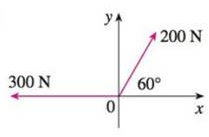Chapter 12.2, Problem 33E

Chapter
Section
Textbook Problem

# Find the magnitude of the resultant force and the angle it makes with the positive x-axis.33.To determine

To find: The magnitude of resultant force (|F|) and angle with positive x-axis (θ) .

Explanation

Given:

The magnitude of first vector as 200 N and a angle with positive x-axis as 60° and the magnitude of second vector as 300 N and angle with positive x-axis as 180° .

Formula:

Write the expression for vector in terms of horizontal and vertical components.

F=|F|cosθi+|F|sinθj (1)

Here,

|F| is magnitude of vector F.

Write the expression for resultant vector of two vectors F1 and F2 (F) .

F=F1+F2 (2)

Write the expression for magnitude of a vector F1i+F2j .

|F|=F12+F22 (3)

Write the expression for angle θ of a vector F, when any of vectors lies in second quadrant.

θ=tan1(F2F1)+180° (4)

Substitute 200N for |F| , and 60° for θ in equation (1),

F1=(200N)cos(60°)i+(200N)sin(60°)j=(200N)(12)i+(200N)(32)j=100i+1003jN

Substitute 300N for |F| , and 180° for θ in equation (1),

F2=(300N)cos(180°)i+(300N)sin(180°)j=(300N)(1)

### Still sussing out bartleby?

Check out a sample textbook solution.

See a sample solution

#### The Solution to Your Study Problems

Bartleby provides explanations to thousands of textbook problems written by our experts, many with advanced degrees!

Get Started

#### Expand each expression in Exercises 122. x(3x+y)

Finite Mathematics and Applied Calculus (MindTap Course List)

#### Find y by implicit differentiation. 38. x3 y3 = 7

Single Variable Calculus: Early Transcendentals, Volume I